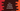# How to convert decimal to binary in C++### Decimal to binary conversion in C++:

In this post, we will learn how to convert one decimal number to binary. Decimal number system is based on 10 and binary number system is based on 2. Our program will take one number as input from the user i.e. a decimal number and print out its binary representation.

There is a simple process to convert one decimal to binary. It is known as repeated division by 2. i.e. we will divide the decimal number by 2 until no further division is possible.

### Example of decimal to binary conversion:

Suppose, we want to convert 19 to binary. Following are the steps required for that:

• 19/2, remainder = 1, quotient = 9
• 9/2, remainder = 1, quotient = 4
• 4/2, remainder = 0, quotient = 2
• 2/2, remainder = 0, quotient = 1
• 1/2, remainder = 1, quotient = 0

We are dividing the value of quotient by 2 in each step until it is 0.

So, the binary representation of 19 is 10011, we are taking all the remainders from last to first.

### C++ program:

``````#include <iostream>

using namespace std;

long decimalToBinary(int decimal){
long binary = 0;
int multiplier = 1;

while (decimal!=0)
{
int reminder = decimal%2;
decimal /= 2;
binary += reminder*multiplier;
multiplier *= 10;
}
return binary;
}

int main()
{
int decimal;
long binary;

cout<<"Enter the decimal value"<<endl;
cin>>decimal;

binary = decimalToBinary(decimal);
cout<<"Binary representation :"<<binary<<endl;

return 0;
}``````

### Explanation:

In this example,

• decimalToBinary method is used to convert one decimal value to binary
• We are asking the user to enter the decimal value and storing it in decimal variable.
• decimalToBinary returns the binary representation in long format. We are storing it in binary variable and printing out the value.
• Inside decimalToBinary, we are keep dividing the value decimal until it become 0. We are getting the remainder, adding it to the variable binary and changing it to decimal/2.
• Once the while loop ends, we are returning the value of binary.

### Sample Output:

``````Enter the decimal value
19
Binary representation :10011``````